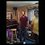# Help: Solution to $y' + 2x^2y = x^2$

Hi, I have been going through the courses for differential equations and just got done with the section on First Order DE. I decided to practice a few more problems on my own and I have been stumped for a while now because when I tried solving it I get different results and I cannot figure out what I am doing wrong. I think it's going to end up being such a small detail I missed but I cannot see it.

I was trying to solve it the way I saw here on Brilliant for a nonhomogeneous equation using the formula $y(x) = A(x)e^{-\int p(x)dx}$ where $A(x) = \int q(x)e^{\int p(x)dx}$ . When I solve for $A(x)$ I get $A(x) = \int x^2e^{\frac{2}{3}x^3}dx$ then after u-substitution I get $A(x) = \frac{1}{2}e^{\frac{2}{3}x^3}$. Going back to the other formula $y(x) = A(x)e^{-\int p(x)dx}$ then means $y(x) = \frac{1}{2}e^{\frac{2}{3}x^3}e^{-\frac{2}{3}x^3}$ which is $\frac{1}{2}$ which is not correct. The way the book does it is by seperation of variables but what am I doing wrong with using the above formulas?Note by Christian Bracamontes
3 years, 1 month ago

This discussion board is a place to discuss our Daily Challenges and the math and science related to those challenges. Explanations are more than just a solution — they should explain the steps and thinking strategies that you used to obtain the solution. Comments should further the discussion of math and science.

When posting on Brilliant:

• Use the emojis to react to an explanation, whether you're congratulating a job well done , or just really confused .
• Ask specific questions about the challenge or the steps in somebody's explanation. Well-posed questions can add a lot to the discussion, but posting "I don't understand!" doesn't help anyone.
• Try to contribute something new to the discussion, whether it is an extension, generalization or other idea related to the challenge.

MarkdownAppears as
*italics* or _italics_ italics
**bold** or __bold__ bold
- bulleted- list
• bulleted
• list
1. numbered2. list
1. numbered
2. list
Note: you must add a full line of space before and after lists for them to show up correctly
paragraph 1paragraph 2

paragraph 1

paragraph 2

[example link](https://brilliant.org)example link
> This is a quote
This is a quote
    # I indented these lines
# 4 spaces, and now they show
# up as a code block.

print "hello world"
# I indented these lines
# 4 spaces, and now they show
# up as a code block.

print "hello world"
MathAppears as
Remember to wrap math in $$ ... $$ or $ ... $ to ensure proper formatting.
2 \times 3 $2 \times 3$
2^{34} $2^{34}$
a_{i-1} $a_{i-1}$
\frac{2}{3} $\frac{2}{3}$
\sqrt{2} $\sqrt{2}$
\sum_{i=1}^3 $\sum_{i=1}^3$
\sin \theta $\sin \theta$
\boxed{123} $\boxed{123}$